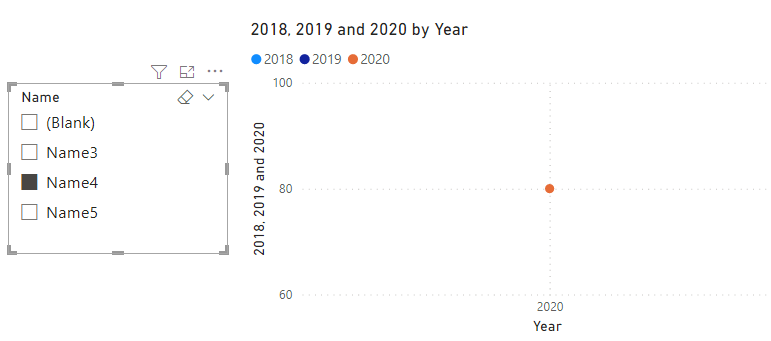cancel
Showing results for
Did you mean:New Member

## Turn into "blank" if no value

Hi all,

I am very new to PowerBI and am searching for your help on the below issue.

The below visual is dragged from 5tables (2018 - 2022), I filter by name of person. With some people, they do not have the data in 2018 or 2019 or 2020... - However the visual keeps shows global data rather than blank.

How can I sort it out please? Thank you.1 ACCEPTED SOLUTIONCommunity Support

Hi, @alp_upgirl

Sample data:Name5 has no data in the 2018 and 2019 tables and should be blank for hours.Measure:

``2018 = CALCULATE(SUM('2018'[Value]),FILTER('2018',[Name]=SELECTEDVALUE('2018'[Name])),FILTER('Date',[Year]=SELECTEDVALUE('Date'[Year])))``
``2019 = CALCULATE(SUM('2019'[Value]),FILTER('2019',[Name]=SELECTEDVALUE('2019'[Name])),FILTER('Date',[Year]=SELECTEDVALUE('Date'[Year])))``
``2020 = CALCULATE(SUM('2020'[Value]),FILTER('2020',[Name]=SELECTEDVALUE('2020'[Name])),FILTER('Date',[Year]=SELECTEDVALUE('Date'[Year])))``

Result:Best Regards,

Community Support Team _Charlotte

If this post helps, then please consider Accept it as the solution to help the other members find it more quickly.

2 REPLIES 2New MemberCommunity Support

Hi, @alp_upgirl

Sample data:Name5 has no data in the 2018 and 2019 tables and should be blank for hours.Measure:

``2018 = CALCULATE(SUM('2018'[Value]),FILTER('2018',[Name]=SELECTEDVALUE('2018'[Name])),FILTER('Date',[Year]=SELECTEDVALUE('Date'[Year])))``
``2019 = CALCULATE(SUM('2019'[Value]),FILTER('2019',[Name]=SELECTEDVALUE('2019'[Name])),FILTER('Date',[Year]=SELECTEDVALUE('Date'[Year])))``
``2020 = CALCULATE(SUM('2020'[Value]),FILTER('2020',[Name]=SELECTEDVALUE('2020'[Name])),FILTER('Date',[Year]=SELECTEDVALUE('Date'[Year])))``

Result:Best Regards,

Community Support Team _Charlotte

If this post helps, then please consider Accept it as the solution to help the other members find it more quickly.